Next: The Periodic Table Up: Spin Previous: Degeneracy

#### Pertubations and Fine Structure

Bohr's formula for the energy levels is quite simple: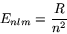This simplicity stems from the simplicity of the formula he used for the energy: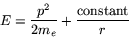where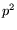is the square of the momentum,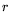is the radial distance from the proton to the electron, and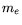is the mass of the electron. The first term represents the kinetic energy, the second the potential energy due to the inverse square force (Coulomb potential). The quantum theorists came along later and said that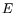,, andare all really operators, and the energy levels are really eigenvalues, but the source of the simplicity remains the same. Simplicity'' is too vague a word: symmetry'' serves better, as we will see.

For example, the formula foris spatially symmetric under rotations about the proton (becauseandare unchanged by such rotations). Thus the formula for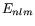cannot depend on, which specifies the orientation of the orbit. If we impose a magnetic field along the-axis, say, thenacquires a new term (of the form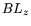, whereis the magnetic field strength, and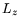is the-component of orbital angular momentum). The new formula fornow depends on, sinceis not symmetric under arbitrary rotations. The magnetic field is referred to as a perturbation.We can see the effects of the magnetic field in changes to the term scheme: some states move up in energy, some move down. Reduced symmetry has lead to reduced degeneracy. Transitions that formerly had the same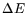now have slightly different's (see figure 0). This means that a single spectral line with no magnetic field will split into multiple spectral lines when the field is turned on. This is the famous Zeeman effect.

Another example: in 1916, Sommerfeld replaced Bohr's simple formula forwith its relativistic equivalent. This lead to a new formula for, depending on both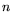and(but not on, of course). These relativistic corrections (or perturbations) account for the so-called fine structure of the hydrogen spectrum, known already to spectroscopists long before 1916.

A historical footnote: one modern author has written:

When Dirac developed relativistic quantum mechanics, the relativistic Coulomb problem proved to be exactly solvable ...But the resulting formula for the energy levels was truly a surprise: The new answer was precisely the old Sommerfeld formula!

How could this possibly be? Clearly Sommerfeld's methods were heuristic (Bohr quantization rules), out-dated by two revolutions (Heisenberg-Schrödinger nonrelativistic quantum mechanics and Dirac's relativistic quantum mechanics) and his methods obviously had no place at all for the electron spin ...So Sommerfeld's correct answer could only be a lucky accident, a sort of cosmic joke at the expense of serious minded physicists.
The author calls this the Sommerfeld puzzle'', and resolves it, but I will discuss it no further.Next: The Periodic Table Up: Spin Previous: Degeneracy